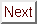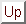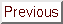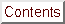Next: Microscopic Models of Up: Experimental Results: Angular Previous: Type II/1 Superconductivity

## Tinkham's Formula Fits to Hc2(theta) in C4KHg

Another peculiarity of the C4KHg Hc2(theta) curves is that they are well-fit by Tinkham's Hc2(theta) formula for thin films:The Tinkham formula fit is decent (cal R = 0.83 at t = 0.29 for a Tc = 1.54 K sample) even without allowing for type I behavior, and is quite good (cal R = 0.26) if type I behavior is taken into account. A summary of the residuals for fits to the Hc2(theta) data is given in Table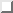, and a direct comparison between the two types of fits is shown in Figurea). Figureb) shows the errors of the two fits as a function of the angle theta. For the Tc = 1.5 K GIC's the Tinkham formula fits have residuals about half of those of the best AGL fits. Consultation of standard tables on statistics shows that (for 39 degrees of freedom and a 3-parameter fit, the conditions of Figure) there is about an 80% probability that the Tinkham formula is a better description of the data than the AGL model. The data on the lower- Tc samples are still best fit by the plain two-parameter AGL theory, just as was reported by Iye and Tanuma.

The reason that a good fit to Eqn.is unexpected is that its derivation requires the assumption that the superconducting order parameter doesn't vary along the direction perpendicular to the specimen's surface. This assumption seems reasonable for a film which has a thickness less than a superconducting coherence length, but is hard to believe when the sample is 105 coherence lengths in thickness. Only if the sample were so disordered along the c-axis that its structural coherence length was on the order of the superconducting coherence length could one envision that the Tinkham formula should apply. However, the metallic nature of the c-axis conductivity and the reproducibility of the Hc2(theta) results from one sample to another argue strongly against this interpretation.

Tinkham's formula has been used to fit Hc2(theta) curves in the artificially structured superlattice superconductors, such as Nb/Cu and Nb/Ta. However in these systems Eqn.fits only below the 3D-2D crossover point (see Chapter), and C4KHg is clearly well into the 3D regime, where Eqn.is supposed to be applicable. The 3D nature of superconductivity in C4KHg is unquestionable because the Klemm-Luther-Beasley r-parameter is about 2000 at 0 K, while 1 is the critical value for the dimensionality crossover. (The question of dimensionality crossover as it relates to GIC's is discussed further in Sectionsand.)Table: Residuals for fits to Hc2(theta) using the AGL formula and the Tinkham formula, both with and without type I behavior. The residual index cal R is defined in Eqn., the AGL formula is Eqn., and the Tinkham formula is Eqn.. Eqn.shows how each of these formulae was modified to account for possible type I superconductivity.Figure: Comparison of the Tinkham formula and AGL theory fits to Hc2(theta) data on a Tc = 1.5 K C4KHg-GIC. bullet, data at t = 0.55. circ, AGL fit with Hc2(0°) = 19 Oe, 1/epsilon = 15.5, and a residual cal R = 0.84. diamond, TF fit with Hc2(0°) = 23 Oe, 1/epsilon = 13, Hc = 41 Oe, and cal R = 0.47. Below, a plot of the errors of each fit versus theta. The same symbols are used.

Despite the statistically significant improvement that Tinkham's formula gives over the AGL Eqn., it is quite hard to justify the use of Tinkham's formula theoretically. One obvious possibility is that the superconductivity measured in C4KHg is not a bulk phenomenon, but is merely due to a thin layer on the surface. The idea is not that there is surface-nucleated superconductivity at the edge of a homogeneous bulk superconductor, but that there could be a second crystallographic phase stable only near the surface. This explanation is appealing because it would help explain the mystery as to the difference between the gold and pink phases of C4KHg : the gold lower- Tc phase which is well-fit by Eqn.would be due to bulk superconductivity, whereas the pink higher- Tc phase would be present only on the surface. A surface phase present only in a thin layer could easily mimic two-dimensional behavior, and would be expected to fit Tinkham's formula.

Superconductivity in a surface layer has already been shown to give good agreement with Tinkham's formula. In lead films doped with thallium, the ``thin film'' was a surface-nucleated layer about a coherence length thick. Nonetheless the ``film'' had critical fields which agreed quite well with the Hc2(theta) shape predicted by Eqn.. Surface superconductivity cannot be responsible for the Hc2(theta) behavior here since it can give only the anisotropy ratio 1.69 = Hc3/ Hc2.

There is one major problem with the idea of the pink ( Tc = 1.5 K) phase of C4KHg being present only as a surface film. That problem is that if thin films are responsible for the angular dependence of Hc2, they should givewhere d is the thickness of the film. As discussed in Section, thin films have a parallel critical field with a temperature dependence of the form Hc2, _|_ ^c propto (1 - t)1/2, a form which is in definite conflict with the linear behavior found for Hc2, _|_ ^c(t). (The temperature dependence of Hc2 at constant angle is discussed in detail in the Section.)

For a given anisotropy, the primary difference between Tinkham's formula and Eqn.is that the Tinkham's formula curve has upward positive curvature for all angles, whereas the AGL angular dependence has an inflection point in the wings of the peak, and has negative curvature at theta = 90°. The challenge in justifying the application of Tinkham's formula to GIC's is to think of a factor that could cause Hc2(theta) to rise more steeply than the AGL dependence. One aspect of the problem that is completely overlooked in the derivation of Eqn.(and in the derivation of Tinkham's formula, for that matter) is the microscopic physics of the flux-line lattice (FLL). In an isotropic superconductor, in the absence of defects, the axis of symmetry of a vortex must lie along the applied field. If the symmetry axis of the vortex were not along the applied field, the screening currents in the vortex would have to be larger, which would cost kinetic energy. Tilley, one of the originators of the AGL model, calculated the properties of the flux-line lattice (FLL) in anisotropic superconductors. He found that the energy of the FLL is lowest when the applied field is along a crystallographic symmetry direction. When the applied field is at an arbitrary angle theta with respect to the symmetry axes, the vortices pay a potential energy price for their misorientation with respect to the crystalline axes. Perhaps when the applied field is oriented only slightly off a crystallographic symmetry direction (such as vecH _|_ ^c), the flux-line lattice might actually minimize its total energy (kinetic energy from screening currents plus potential energy of misorientation) by orienting the vortices' symmetry axes along the crystallographic symmetry direction rather than along the applied field. Quantitative calculations by Kogan and Kogan and Clem suggest that this rotation of the flux-line lattice may be a common feature of anisotropic superconductors. It is proposed that rotation of the FLL to the crystallographic symmetry direction for theta near 90° could help explain why the experimental data rise more sharply than the AGL model in the wings of the Hc2(theta) peak. Unfortunately, coming up with a test of this hypothesis is not easy. Magnetic torque measurements might be able to give some information. Kogan and Clem suggest neutron scattering and nuclear magnetic resonance tests.

In summary, Tinkham's formula provides the best fit to the Hc2(theta) data for the Tc = 1.5 K specimens. However, the assumptions used in the derivation of the Tinkham formula imply a temperature dependence of Hc2 which is strongly in conflict with experimental data. Therefore, solving the Hc2(theta) problems of the C4KHg data with Tinkham's formula creates new problems for Hc2(t). Figureb) shows that the Tinkham's formula fit seems to be an improvement on the AGL theory principally in the wings, where the angular dependence of the demagnetization may play a role. Therefore an unsatisfying but reasonable conclusion is that the agreement with Tinkham's formula is fortuitous, implying that the formula somehow mimics the combination of anisotropy, tilt, type I behavior, demagnetization effects and mosaic spread that were actually present in the experiments. Alternatively, the microscopic details of the flux-line lattice may be having an effect on Hc2(theta).Next: Microscopic Models of Up: Experimental Results: Angular Previous: Type II/1 Superconductivity

alchaiken@gmail.com (Alison Chaiken)
Wed Oct 11 22:59:57 PDT 1995